Courses

# Electromagnetic & Analog Communications - MCQ Test - 1

## 15 Questions MCQ Test GATE ECE (Electronics) 2022 Mock Test Series | Electromagnetic & Analog Communications - MCQ Test - 1

Description
This mock test of Electromagnetic & Analog Communications - MCQ Test - 1 for Electronics and Communication Engineering (ECE) helps you for every Electronics and Communication Engineering (ECE) entrance exam. This contains 15 Multiple Choice Questions for Electronics and Communication Engineering (ECE) Electromagnetic & Analog Communications - MCQ Test - 1 (mcq) to study with solutions a complete question bank. The solved questions answers in this Electromagnetic & Analog Communications - MCQ Test - 1 quiz give you a good mix of easy questions and tough questions. Electronics and Communication Engineering (ECE) students definitely take this Electromagnetic & Analog Communications - MCQ Test - 1 exercise for a better result in the exam. You can find other Electromagnetic & Analog Communications - MCQ Test - 1 extra questions, long questions & short questions for Electronics and Communication Engineering (ECE) on EduRev as well by searching above.
QUESTION: 1

Solution:
QUESTION: 2

### An AC voltage source v = Vo sin cot is connected across a parallel plate capacitor 'C'. The displacement current in the capacitor is equal to

Solution:

Conduction current inThe connecting wires

= i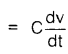= CωVo cos ωt   .............. (I)

For a parallel plate capacitor of area 'A and plate separation 'd',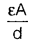......................(ii)

Electric field (E) =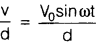Also,              D = Electric flux density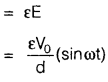Now, displacement current (id)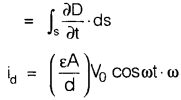= CωVo cos ωt   ............  (iii)

From equation (i) and (iii) we have

i = id

QUESTION: 3

### A plane wave is characterized by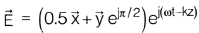The wave is

Solution:
QUESTION: 4

Skin depth at a frequency of 1.6 MHz inaluminium, σ = 38.2, μr = 1.

Solution:

Skin depth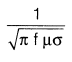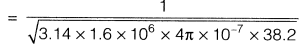= 64.4 mm

QUESTION: 5

An uniform sinusoidal plane wave in air hasthe following expression for electric field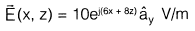The frequency of operation will be

Solution:

General expression,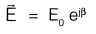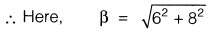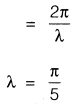Now, frequency =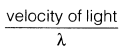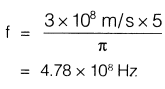QUESTION: 6

A dipole antenna was radiating with some excitation in free space radiating a certain amount of the power. If this antenna is immersed in a lake where water is non­magnetic and non-dissipative but has a dielectric constant of 81, the radiated power with the same excitation will

Solution:
QUESTION: 7

A dipole antenna of λ/8 length has anequivalent total loss resistance of 1.5ΩThe efficiency of the antenna is:

Solution:
QUESTION: 8

Match List-I (Laws) with List-II (Applica- tions) and select the correct answer:

List-I

A. Ampere's law

B. Biot's law

C. Coulomb's law

D. Gauss's law

List-II

To find the

1. force on a charge

2. force due to a current carrying

3. electric flux density at a point

4. magnetic flux density at a point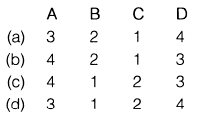Solution:
*Answer can only contain numeric values
QUESTION: 9

A signal m(t) is band limited upto 20 kHz is sampled at `fs' kHz. An ideal LPF having cut off frequency 57 kHz is used to reconstruct the m(t). The minimum value of `fs' required to reconstruct m(t) without distortion is,__________   kHz

Solution:

For the distortion less reconstruction of m(t)

signal,

fs - fm ≥ 57 kHz

⇒   fs ≥ 57 + fin
⇒   fs  ≥  77 kHz

QUESTION: 10

An AM transmitter is to deliver a total power of 100 W to an antenna. The percentage modulation is to be 100%. The power contained in each sideband is

Solution: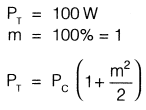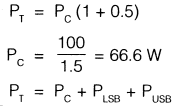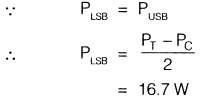QUESTION: 11

Which one of the following sequence is correct regarding bandwidth (BW) of various analog modulated system?

Solution:
QUESTION: 12

The nyquist sampling rate for the signal s(t) = 20 cos(50πt) . cos2(150πt) is,

Solution: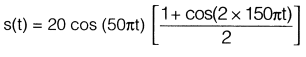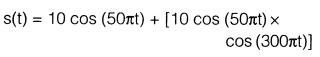The maximum frequency component will be

= 150 + 25

= 175 Hz

∴ Nyquist sampling rate

= fs

= 2 fm

= 2 x 175Hz

= 350 samples/sec

*Answer can only contain numeric values
QUESTION: 13

The modulation index of an FM signal having a carrier swing of 100 kHz and modulating frequency of 8 kHz is

Solution:

∴ Carrier swing = 2 x Δf

⇒  Δf = 50 kHz

Also, modulation index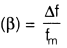∴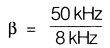= 6.25

QUESTION: 14

For the generation of an AM signal we have m(t) = cos (4π x 104 t) and c(t) = 5 cos(2π x 106 t) where m(t) represents message signal and c(t) represents carrier signal then what would be the relation between total power (PT) and power contained in one of its side band (Ps) if the modulation index is 0.5?

Solution:

∴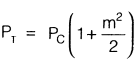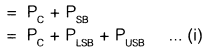Let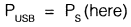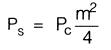⇒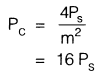.       .......(ii)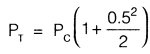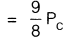∴ From equation (ii)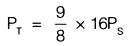⇒ PT = 18 Ps

QUESTION: 15

Percentage modulation of an AM wave having a power content of 8 kW at carrier frequency and 2 kW in each of its bands is given by

Solution:

Given That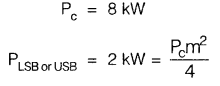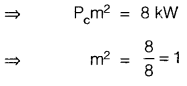⇒ m= 1

∴ % modulation = 100%